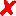## total (pooled) variance: examples [Power / Sample Size]

Dear Nav!

❝ […] But how would you get the inter-subject variability from the ANOVA for parallel design in order to calculate the sample size.

Some more references and explanations.
In a parallel design we have no direct access to inter-subject variability, what we see is the total (sometimes called pooled) variability.

From a 2×2×2 crossover design we may estimate
• intra-subject variability as
$$CV_e(\%)=100\sqrt{e^{MS_e}-1}$$

• inter-subject variability as
$$CV_s(\%)=100\sqrt{e^{(MS_s{\color{Red} -}ME_e)/2}-1}$$

• total (pooled) variability as
$$CV_p(\%)=100\sqrt{e^{(MS_s{\color{Red} +}ME_e)/2}-1}$$
For details see
1. Midha KK, Ormsby ED, Hubbard JW, McKay G, Hawes EM, Gavalas L, McGilveray IJ. Logarithmic Transformation in Bioequivalence: Application with Two Formulations of Perphenazine. J Pharm Sci. 1993;82(2):138–44.
2. Hauschke D, Steinijans VW, Diletti E. Presentation of the intrasubject coefficient of variation for sample size planning in bioequivalence studies. Int J Clin Pharmacol Ther. 1994;32(7):376–8.
So if you only have data from a parallel study, your variability is the total (pooled) one, not the inter-subject variability. People dealing with parallel studies sloppily talk about inter-subject variability instead of total.1 So I would guess you may simply use formulas and software intended for inter-subject CV (although you have the total CV). At least it’s a conservative approach (in other words, you will only loose some money because your study will be slightly overpowered)

❝ Re: 60% of inter to estimate the intra: I am a statistician and I routinely see the PK individuals taking 60% of inter to be the intra for estimating sample size for a crossover design and always wondered about the validity of the approach.

I give you two examples from my 2×2 cross-over studies (for low/medium/high intra-subject CV) to get an impression about the lacking relationship of CV-intra/-inter/-total:2

Methyphenidate 20 mg MR single dose sprinkled, AUCt, n=12
CV-intra  7.00% [=36% of inter, 34% of total]CV-inter 19.1%
CV-total 20.4%

Paroxetine 30 mg IR steady state, AUCtau, n=32
CV-intra 25.2% [=46% of inter, 41% of total]CV-inter 55.1%
CV-total 62.1%

Lansoprazole 30 mg single dose fasting, Cmax, n=47
CV-intra 47.0% [=187% (!) of inter, 86% of total]CV-inter 25.1%
CV-total 54.6%

I can’t see this rule of thumb of ‘CV-intra = 60% CV-inter’ to be applicable …

1. Intra-subject variability does not ‘disappear’ just because you made only one observation.

Dif-tor heh smusma 🖖🏼 Довге життя Україна!Helmut SchützThe quality of responses received is directly proportional to the quality of the question asked. 🚮
Science QuotesIng. Helmut Schütz